# Study Guide

## Core Academic Skills Assessment (CASA) Sample Multiple-Choice Questions

### Mathematics

The following reference material will be available to you during the test:

##### Objective 0005Number and Quantity (Standard 9)

1. A shopper's entire purchase included 2 neckties that cost \$12.75 each, a shirt that cost \$22.00, and a pair of shoes that cost \$45.50. If the sales tax on the entire purchase was 6%, how much change did the shopper receive from a \$100 bill?

1. \$1.42
2. \$3.26
3. \$4.60
4. \$6.74
Correct Response: A.
This question requires the examinee to demonstrate the ability to solve multistep problems using a variety of number representations. The cost of the items purchased is found by 12.75 × 2 + 22.00 + 45.50 = 93.00. The purchase plus the tax is found by 93.00 + (93.00 × 0.06) = 98.58. If the shopper tenders a \$100 bill, the change is found by \$100.00 – \$98.58 = \$1.42.

2. Use the table below to answer the question that follows.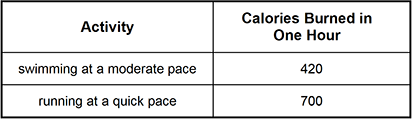A person is using the table as a guide to plan an exercise routine. About how many minutes would the person have to swim at a moderate pace to burn the same number of calories burned by running at a quick pace for 24 minutes?

1. 14 minutes
2. 36 minutes
3. 40 minutes
4. 50 minutes
Correct Response: C.
This question requires the examinee to demonstrate the ability to apply ratio concepts and proportional reasoning to solve real-world problems. The number of calories burned by running for 24 minutes is found by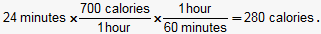The number of minutes that a person needs to swim to burn 280 calories is found by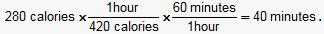##### Objective 0006Algebra and Functions (Standard 10)

3. Solve 1.5(y + 4) = 7.5y – 9 for y.

1. y = 1.4
2. y = 1.7
3. y = 2.2
4. y = 2.5
Correct Response: D.
This question requires the examinee to demonstrate the ability to solve one-variable equations. Since 1.5(y + 4) = 7.5y – 9, then by the distributive property 1.5y + 6 = 7.5y – 9. By adding 9 to and subtracting 1.5y from both sides of the equation as follows, 1.5y – 1.5y + 6 + 9 = 7.5y – 1.5y – 9 + 9 means 15 = 6y and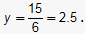##### Objective 0007Measurement and Geometry (Standard 11)

4. Use the diagram below to answer the question that follows.If the two pentagons shown are similar, what is the measure of side M?

1. 3
2. 4
3. 5
4. 6
Correct Response: B.
This question requires the examinee to demonstrate the ability to apply the concept of similarity. Since the two pentagons are given as similar, then corresponding sides of the pentagons form ratios that are equal to each other, such as: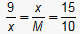. Using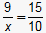and cross multiplying means 15x = 90 and x = 6. Since x = 6, then. Again, by cross multiplying 15M = 60 and M = 4.

##### Objective 0008Statistics and Probability (Standard 12)

5. Use the graph below to answer the question that follows.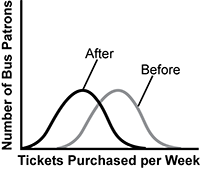A small city's transit company has made significant changes to its bus routes. Weekly bus ticket sales before and after making the changes are displayed in the graph. The distributions in the graph show that the changes made to bus routes produced which of the following outcomes?

1. The standard deviation of bus ticket sales has decreased.
2. Bus patrons seem to be buying fewer tickets.
3. The standard deviation of bus ticket sales has increased.
4. Bus patrons seem to be buying more tickets.